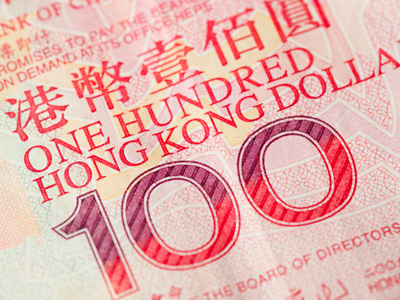When multiplying decimals by 100 the digits move two places to the left.

# Level 3-4 Numbers - Decimals - Powers of 10

Decimal numbers are those that are smaller than a one and written after a decimal point. Decimal numbers are an alternative way of writing fractions. A quarter written as a fraction would be 14 but written as a decimal would be 0.25. This KS3 Maths quiz will help you understand multiplying and dividing decimals by the powers of 10, like 10, 100 or 1,000.

When you multiply decimal numbers by 10 all the digits move one place to the left. Divide by 10 and they all move one place to the right. In a similar way, when you multiply decimal numbers by 100 all the digits move two places to the left. Divide by 100 and they all move two places to the right. Remember that rule and you'll soon get to grips with multiplying and dividing decimals.

Multiplying or dividing decimals by 10, 100 or even 1,000 is really quite easy once you get the hang of it. This quiz will help you do just that. Take your time and read each question carefully before submitting your answer. See if you can get 10 out of 10 with the powers of 10 and decimals.

1.
What is 7.75 x 100?
0.775
0.7
77.5
775
To multiply by 100 move the digits two places to the left
2.
What is 4.65 x 10?
0.465
46.5
465
4,650
Just shift the digits one place to the left
3.
What is 44.87 x 100?
4.4
449
4,487
4,490
To multiply by 100 move the digits two places to the left
4.
What is 88.3 / 100?
0.883
8.083
8.83
883
Bear in mind the digits move, not the decimal point
5.
What is 0.04 / 10?
0.004
004
00.4
4
Note that you need to add a zero before the decimal point
6.
What is 0.08 x 10?
0
0.1
0.8
8
Remember it's the digits that move one place to the left when multiplying by 10
7.
When dividing decimals by 10, how many places do the digits move?
0
1
2
3
1 zero means move 1 place
8.
What is 68.72 / 10?
6
6.872
687.2
6,872
Just shift the digits one place to the right
9.
When multiplying decimals by 100, how many places do the digits move?
0
1
2
3
2 zeros before the decimal means move 2 places
10.
What is 692.14 / 100?
0.69214
6.9214
69.214
6,921.4
To divide by 100 move the digits two places to the right